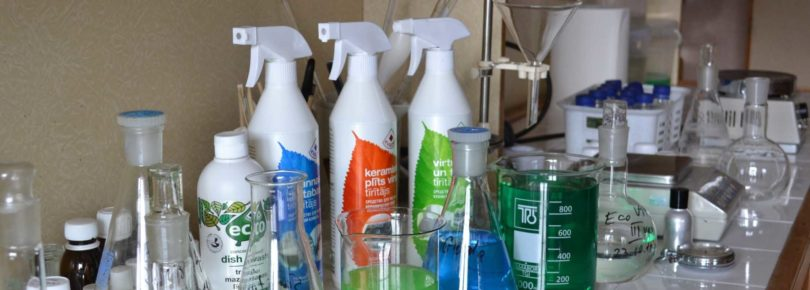# Chemical Analysis Questions and Answers### Chemical Analysis Practice Problems:

Ques. Nitrates of all the metals are
(a) Coloured
(b) Unstable
(c) Soluble in water
(d) Insoluble in water

Ans. (c)

Ques. Pb++, Cu++, Zn++ and Ni++ ions are present in a given acidic solution. On passing hydrogen sulphide gas through this solution the available precipitate will contain
(a) PbS and NiS
(b) PbS and CuS
(c) CuS and ZnS
(d) CuS and NiS

Ans. (b)

Ques. The number of hydroxide ions, produced by one molecule of sodium carbonate (Na2CO3) on hydrolysis is
(a) 2
(b) 1
(c) 3
(d) 4

Ans. (a)

Ques. In acidic medium, dichromate ion oxidises ferrous ion to ferric ion. If the gram molecular weight of potassium dichromate is 294 grams, its gram equivalent weight is……grams
(a) 294
(b) 127
(c) 49
(d) 24.5

Ans. (c)

Related: Calorimetry Problems

Ques. When H­­2S is passed through in II group sometimes solution becomes milky. It indicates the presence of
(a) Acidic salt
(b) An oxidising agent
(c) Thiosulphate
(d) A reducing agent

Ans. (b)

Ques. Metallic tin in the presence of HCl is oxidise K2Cr2O7 to stannic chloride. What volume of decinormal dichromate solution would be reduced by 1 g of tin
(a) 168.49 ml
(b) 175.49 ml
(c) 170.50 ml
(d) 162.38 ml

Ans. (a)

Ques. H2S will precipitate the sulphides of all the metals from the solution of chlorides of Cu, Zn and Cd, if
(a) The solution is aqueous
(b) The solution is acidic
(c) The solution is dilute acidic
(d) Any of these solutions is present

Ans. (c)

Ques. 50 ml 10 N – H2SO4, 25 ml 12N–HCl and 40 ml 5 N –  HNO3 were mixed together and the volume of the mixture was made 1000 ml by adding water. The normality of the resultant solution will be
(a) 1 N
(b) 2 N
(c) 3 N
(d) 4 N

Ans. (a)

Ques. Sodium carbonate cannot be used in place of ammonium carbonate for the precipitation of the fifth group radicals because
(a) Sodium ions interfere with the detection of fifth group radicals
(b) Concentration of carbonate ions is very low
(c) Sodium will react with acidic radicals
(d) Magnesium will be precipitated

Ans. (d)

Related: Capacitor questions

Ques. An aqueous solution of colourless metal sulphate M, gives a white precipitate with NaOH. This was soluble in excess of NaOH. On passing H2S through this solution a white precipitate is formed. The metal M in the salt is
(a) Ca
(b) Ba
(c) Al
(d) Zn

Ans. (d)

Ques. Which of the following is soluble in yellow ammonium sulphide
(a) CuS
(b) CdS
(c) SnS
(d) PbS

Ans. (c)

Ques. A compound is soluble in water. If ammonia is added, a red precipitate appears which is soluble in dilute HCl. The compound has
(a) Aluminium
(b) Zinc
(c) Iron

Ans. (c)

Ques. The equivalent weight of KMnO4 in alkaline medium will be
(a) 31.60
(b) 52.66
(c) 79.00
(d) 158.00

Ans. (d)

Ques. The metal that does not give the borax-bead test is
(a) Chromium
(b) Nickel
(d) Manganese

Ans. (c)

Ques. The ratio of amounts of H2S needed to precipitate all the metal ions from 100 ml of 1 M AgNO3 and 100 ml of 1 M CuSO4 is
(a) 1 : 2
(b) 2 : 1
(c) Zero
(d) Infinity

Ans. (a)

Ques. 100 cm3 of 0.1 N HCl solution is mixed with 100 cm3 of 0.2 N NaOH  solution. The resulting solution is
(a) 0.1 N and the solution is basic
(b) 0.05 N and the solution is basic
(c) 0.1 N and the solution is acidic
(d) 0.05 N and the solution is acidic

Ans. (b)

Ques. Methyl orange gives red colour in
(a) Sodium carbonate solution
(b) Sodium chloride solution
(c) Hydrochloric acid solution
(d) Potassium hydroxide solution

Ans. (c)